# ML Aggarwal Class 10 Solutions for ICSE Maths Chapter 5 Quadratic Equations in One Variable Ex 5.1

## ML Aggarwal Class 10 Solutions for ICSE Maths Chapter 5 Quadratic Equations in One Variable Ex 5.1

ML Aggarwal Class 10 Solutions for ICSE Maths Chapter 5 Quadratic Equations in One Variable Ex 5.1

Question 1.
Check whether the following are quadratic equations:
(i) $$\sqrt { 3 } { x }^{ 2 }-2x+\frac { 3 }{ 5 } =0$$
(ii) (2x + 1) (3x – 2) = 6(x + 1) (x – 2)
(iii) $${ (x-3) }^{ 3 }+5={ x }^{ 3 }+7{ x }^{ 2 }-1$$
(iv) $$x-\frac { 3 }{ x } =2,x\neq 0$$
(v) $$x+\frac { 2 }{ x } ={ x }^{ 2 },x\neq 0$$
(vi) $${ x }^{ 2 }+\frac { 1 }{ { x }^{ 2 } } =3,x\neq 0$$
Solution: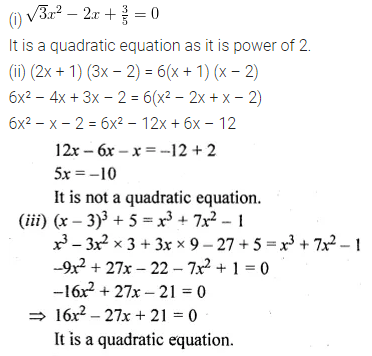Question 2.
In each of the following, determine whether the given numbers are roots of the given equations or not;
(i) x² – x + 1 = 0; 1, -1
(ii) x² – 5x + 6 = 0; 2, -3
(iii) 3x² – 13x – 10 = 0; 5,$$\\ \frac { -2 }{ 3 }$$
(iv) 6x² – x – 2 = 0; $$\\ \frac { -1 }{ 2 }$$, $$\\ \frac { 2 }{ 3 }$$
Solution: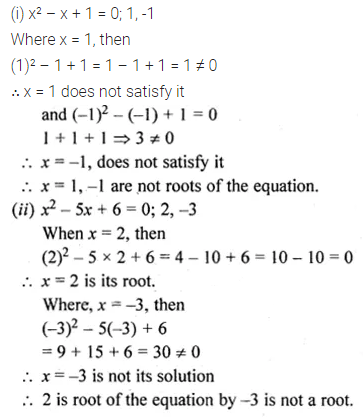Question 3.
In each of the following, determine whether the given numbers are solutions of the given equation or not:
(i) x² – 3√3x + 6 = 0; √3, -2√3
(ii) x² – √2x – 4 = 0, x = -√2, 2√2
Solution: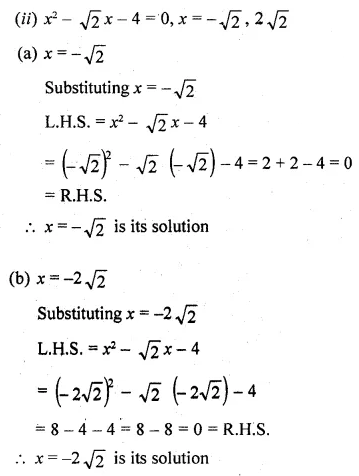Question 4.
(i) If $$– \frac { 1 }{ 2 }$$ is a solution of the equation 3x² + 2kx – 3 = 0, find the value of k.
(ii) If $$\\ \frac { 2 }{ 3 }$$ is a solution of the equation 7x² + kx – 3 = 0, find the value of k.
Solution: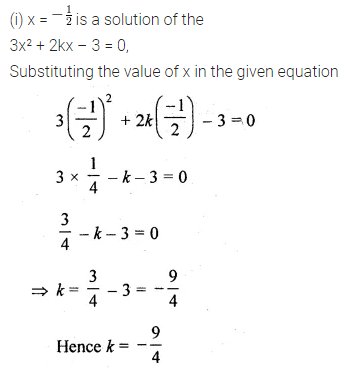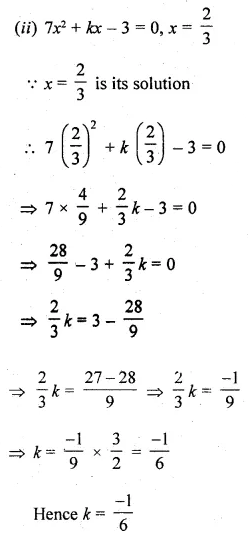Question 5.
(i) If √2 is a root of the equation kx² + √2 – 4 = 0, find the value of k.
(ii) If a is a root of the equation x² – (a + b)x + k = 0, find the value of k.
Solution: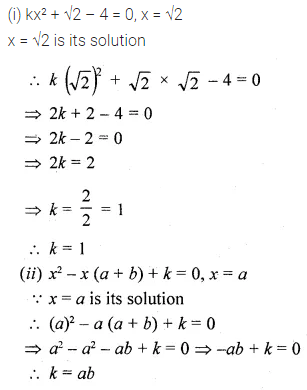Question 6.
If $$\\ \frac { 2 }{ 3 }$$ and -3 are the roots of the equation px² + 7x + q = 0, find the values of p and q.
Solution:ML Aggarwal Class 10 Solutions for ICSE Maths